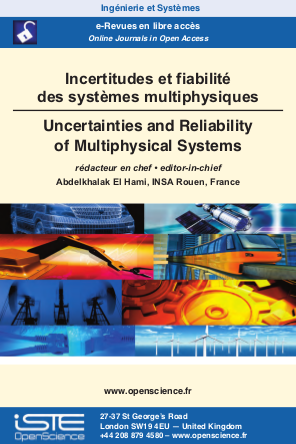# Vol 4 - Issue 1

## List of Articles

Numerical simulation and experimental validation of the air flow around S809 Airfoil for Wind Turbine Blade

Wind energy is one of the oldest sources of energy used by mankind. Today with the negative impact of fossil energy on the environment, the installation of wind farms all over the world is experiencing a considerable boom. To improve its performance and reliability, good modeling of the air flow around a wind turbine blade profile is very decisive. In this article, a numerical simulation of the S809 aerodynamic profile was performed by ANSYS CFX using several models of turbulence and at different angles of attack. Subsequently the calculated pressure coefficients are compared
with experimental data to validate the simulation accuracy and choose the appropriate turbulence model.

An Exploratory Study of the Sheet Hydroforming Process

This article presents an exploratory study of the hydroforming process of a sheet. Before proceeding to the simulations, an experimental test was carried out to validate the mathematical model of simulation by finite elements. An experimental geometrical control makes it possible to determine the shape of the deformation in order to compare it with the simulation looks. The simulation results made it possible to choose the parameters necessary to have a good deformation.

Multiobjective Optimization of NACA0012 Airfoil based Non-dominated Sorting Genetic Algorithm

Multidisciplinary optimization techniques become more and more applied in the field of aerodynamics due to the rapid development of computers high-performance, numerical methods and optimization algorithms. These techniques coupled with Computational Fluid Dynamics (CFD), which aims to incorporate mathematical relations and algorithms to analyze and solve fluid flow problems, involve the use of those numerical methods and algorithms to improve the fluid flow solutions. CFD analysis of an airfoil determines its ability by producing results such as lift and drag forces, and the application of an optimization algorithm involves manipulating these aerodynamic performances according to the requirements. In this work, a numerical investigation, using ANSYS/FLUENT software, of two-dimensional transonic flow over a NACA0012 airfoil was conducted and compared with the provided experimental data. Then a multiobjective optimization algorithm, based on a Non-dominated Sorting genetic algorithm (NSGA-II), was coupled with FLUENT in order to obtain an improved performance control of the aerodynamic coefficients of the airfoil.

Numerical Method of identification of the mechanical and dynamic behavior of materials used in automotive calculation

A coherent and reasonable modeling behavior of the material and its damage under the coupled effects of the deformation, the strain rate and the temperature compared to the stress of flow of the material is remarkably crucial in order to design and optimize the parameters that characterize the material numerically and minimize the maximum error between simulation and experiment. The Johnson-Cook flow constraint model was adopted to model and predict the behavior of material flow at strain rate and temperature average. In addition, the work that has been done in this article is to explain each phase of identification of the parameters of the constructed model and how it is systematically examined in relation to the experimental data. Then, to predict the behavior of damage, the failure model proposed by Johnson and Cook was used to determine the parameters of rupture of the model, based initially on the determination of the triaxiality for an ambient temperature and under a quasi-static strain rate condition. The method explained in this work is the most used in the automotive industry to characterize suitable materials for numerical crash calculation.

Thermal optimization of the high electron mobility transistor (HEMT) using the CMA-ES method

The optimization aims to ensure a robust system design with minimal cost, this work focuses on the optimization of the High Electron Mobility Transistor (HEMT), it is a very important element in high power mechatronic systems. It contains in its structure several layers of materials, the geometrical and thermal parameters of these layers influence the operating temperature of the transistor, hence its performance. Using the CMA-ES method coded on Matlab, a finite element model developed on Comsol multiphysics and a coupling between the two softwares, these parameters have been optimized in order to reduce the maximum operating temperature of the HEMT, so that the transistor performs its function with less influence on the other characteristics.

Modelling of LV distribution network with preassembled Aluminium cables

The increase in the use of preassembled aluminum cables to replace old copper lines requires special attention. In this article, it is a question of establishing the mathematical equations which define the functioning of a network constructed with preassembled aluminum cables. To use this mathematical model, a test network was created, with four cables of 460 IEEE configuration. Our analysis are mainly based on the rate of imbalance and the voltage drops that these lines thus formed can generate. We propose a modified “Forward/Backward” algorithm to execute this analysis. On the test network, two cable layouts were approached, namely a so-called “polygonal” layout and a second so-called “linear” layout. The first layout gives us maximum voltage imbalance rate in 0.0083% and a maximum voltage drop in 1.5%. The second layout gives us very similar results. So, we can consider that the layout has an insignificant impact on lines performances.### Other issues :

2017

Volume 17- 1

Optimization and Reliability
Numéro 2

2018

Volume 18- 2

Issue 1
Issue 2

2019

Volume 19- 3

Issue 1
Issue 2

2020

Volume 20- 4

Issue 1
Issue 2